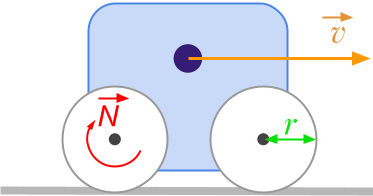Question #107

# How to convert linear speed expressed in meters per second [m/s] to angular velocity expressed in revolutions per minute [rpm]?The formula to convert linear speed expressed in meters per second [﻿﻿] to angular velocity expressed in revolutions per minute [﻿﻿] is given by:

﻿﻿

Considering the units:

﻿﻿

﻿﻿

where:

• ﻿﻿ is the linear velocity expressed in ﻿﻿
• ﻿﻿ i s the angular velocity expressed in ﻿﻿
• ﻿﻿ i s the radius expressed in ﻿﻿

10 events in history

Icons proudly provided by Friconix.